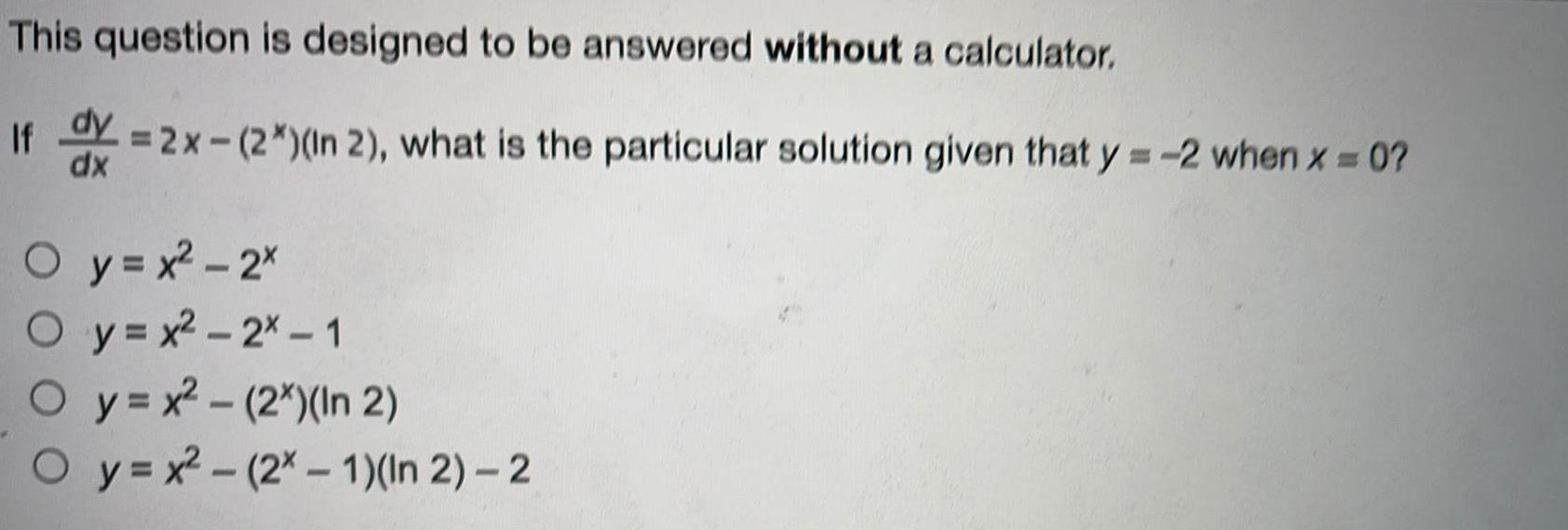Question:

# This question is designed to be answered without aThis question is designed to be answered without a calculator. If dy/dx=2x-(2*) (In 2), what is the particular solution given that y=-2 when x = 0? y=x²-2x y=x²-2x - 1 y=x²-(2x)(In 2) y=x²- (2x - 1)(In 2) - 2